Bottom line: Percentage change formulas require a lot of typing to create a simple calculation.  This macro will create the entire formula for you, including the IFERROR function.

Skill level: Intermediate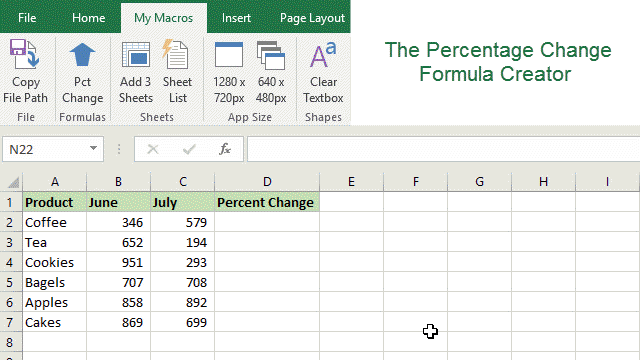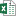Percentage Change Formula Creator Macro (19.0 KB)

## The Percentage Change Formula Explained

To calculate the percentage change between two numbers we typically write a formula like the following:

=(C2-B2)/B2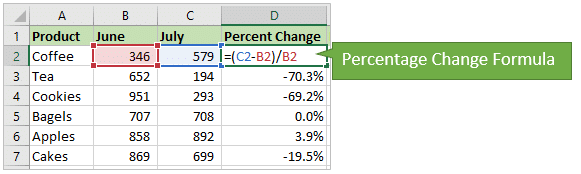Whenever I am creating this formula I always think, “new minus old, divided by old”.  Or:

=(new value – old value) / old value

If we want to handle/prevent any divide by zeros (#DIV/0!), then we can wrap the formula in the IFERROR function.

=IFERROR((C2-B2)/B2,0)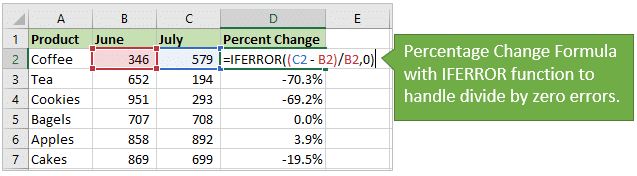The formula above would return a zero if the denomiator (A1/old value) is zero.

This formula can also be used to calculate discount percentages, so you can use it when you are shopping.

discount % = (new discounted price – old regular price) / old regular price

### Simplified Formula

The formula can also be simplified to:

=(new value / old value) – 1

This will return the same result as the other formula.  The formula you use is a matter of personal preference, or your boss's preference. 🙂  Thanks to XLArium for mentioning this is the comments.

Either way, there are a ton of different uses for the percentage change formula. It's one that should be in every Excel user's toolbox.

## The Percent Change Formula Creator Macro

The percentage change formula isn't too terribly long, but it can take some time to type out.  So I wrote this simple macro that will write the formula for us.

Here's how it works…

1. The macro uses the InputBox method, and asks you to select the cells that contain the new and old values.
2. It then writes the percentage change formula, including the IFERROR function, and puts the formula in the ActiveCell.

It's a super simple time saver for a very common formula. 🙂

Here is the VBA code.  You can copy/paste it to your personal macro workbook.

```Sub Percent_Change_Formula()
'Description: Creates a percentage change formula
'Source: https://www.excelcampus.com/vba/percentage-change-formulas-macro/

Dim rOld As Range
Dim rNew As Range
Dim sOld As String
Dim sNew As String
Dim sFormula As String

'End the macro on any input errors
'or if the user hits Cancel in the InputBox
On Error GoTo ErrExit

'Prompt the user to select the cells
Set rNew = Application.InputBox( _
"Select the cell that contains the NEW number", _
"Select New Cell", Type:=8)
Set rOld = Application.InputBox( _
"Select the cell that contains the OLD number", _
"Select Old Cell", Type:=8)

'Get the cell addresses for the formula - relative references
sNew = rNew.Address(False, False)
sOld = rOld.Address(False, False)

'Create the formula
sFormula = "=IFERROR((" & sNew & " - " & sOld & ")/" & sOld & ",0)"

'Create the formula in the activecell
ActiveCell.Formula = sFormula

ErrExit:

End Sub```

It's important to note that this macro will override the value or formula in the activecell with the result.  We can add additional code to prevent that.  More on that below.

## How to Use the Macro

I put this macro in my Personal Macro Workbook, and then create a ribbon button and/or keyboard shortcut for it.  You could also put it in an add-in file.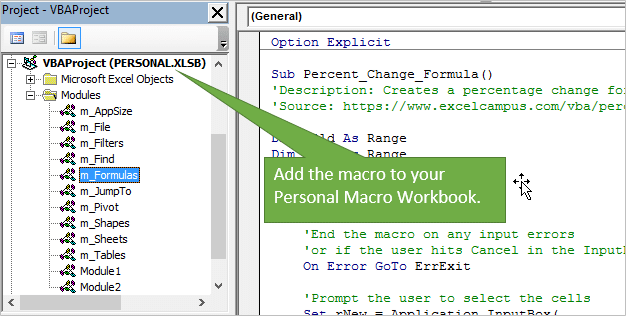Either of these options will allow you to use the macro on any Excel file you have open.  This means that you do NOT have to copy/paste the code to each file you want to use it in.  You can put it in your Personal Macro Workbook, and use it on ANY file.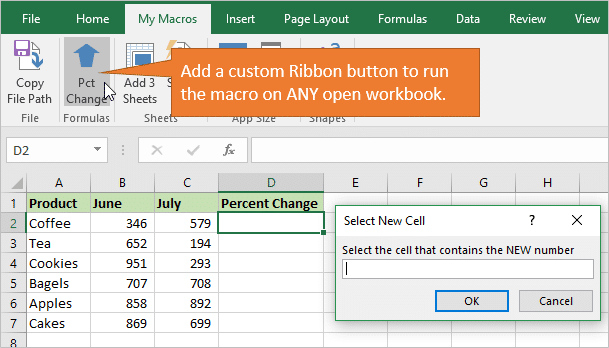Here is a screencast of the macro in action.  You can see how simple it is to create the formula.  All you have to do is click a few buttons and select two cells.  No typing required! 🙂Checkout my article and video series on the Personal Macro Workbook for details on how to set this up and create custom ribbon buttons to run your macros.

## Other Options for the Percentage Change Macro

The possibilities are pretty much endless for this macro.  You can change it to fit your needs.  Here are a few things we could change:

• Change the value_if_error value from 0 (zero) to another value.
• Remove the IFERROR function completely.
• Warn the user before putting the formula in the active cell.
• Copy the formula to the clipboard instead of putting it in the active cell.
• Format the active cell with a percentage format.
Code: ActiveCell.NumberFormat = “0.0%”

What other changes would you like to make?  Please leave a comment below with your suggestions or questions.  Thanks! 🙂

•munidsa says:

how can I adopt above formula to change values in a column by a percentage?(ex: all values in column “a’ to be increased by 9.5%)
database which carries prices has to be changed time to time by a percentage as the market prices are announced.

•Vicky says:

Did you find an answer to this problem?

•Jon Acampora says:

Hi Munidsa,

I believe you are looking for a formula similar to the one in this screenshot.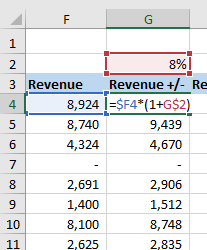This formula multiples the original amount by (1+[% change]). We add 1 to the % change number to increase or decrease the original amount by the % change amount. The % change can be a negative number to decrease the original number.

Notice that cell G2 is an absolute reference. Row 2 is anchored (G\$2), so the formula can be copied down to all cells in the column below.

The user can then just change the value in cell G2 to apply the change to all the cells in the column.

I hope that helps. Thanks again and have a nice day! 🙂

•Sharon says:

Hey,
One of the cells in my Macros spreadsheet says F10% in the formula bar. I’m not sure what this means. Does this mean that the cell’s (here, F10) data is converted to percentage?

•Jon Acampora says:

Hi Sharon,
Yes, that notation can be used when you have a whole number in a cell that you want to convert to a percent. It basically divides the number by 100.

Let’s say you have the number 9 in cell F10. If you were to apply the percent number formatting to cell F10, the result would be 900%. This is because 9 is a whole number.

When you create the formula =F10% in another cell, the result will be 0.09. You can then apply the percent number formatting to this cell to see the result of 9%.

This technique can be used with data sets that output a column of percent values as whole numbers. However, I recommend just dividing the number by 100 instead. It is much easier for others to understand that formula. The % at the end of the reference is weird when you see it for the first time, and usually requires explanation.

I’ll do a follow-up post on this in the future. I hope that helps. Thanks again and have a nice day! 🙂

•Alison says:

There are cases when dividing some small numbers, it will generate -1056783.9% this sort of long percentages. How can I change it to say 100%?

Thanks,
Alison

•PC says:

Hi Jon, This is great! I am looking to create automation with this formula: =IFERROR((AN16-AM16)/AM16,0) for the Percent Change monthly. Your macro worked great, but I still would need to click on the New vs Old cells. How can I modify your VBA code above to automatically calculate a new percent change when new value is entered for the new month w/out selecting the new/old cells. Thanks!

old: =IFERROR((AN16-AM16)/AM16,0)
new: =IFERROR((AO16-AN16)/AN16,0)

•L CODY says:

if the old number was a zero and the new number was a dollar amount how do you show a negative (a loss)

•Roy says:

This was a great lesson for me to understand some of the differences between execution of Excel’s formulas/functions and VBA code. i.e. worksheet vs module

I added some code to the module to format the ActiveCell to “percent”. This was nice because the formatting is also applied after any copy and paste action.

Feeling all proud, I then added more code to change the interior cell colorindex when a “divide-by-zero” event occurred. But quickly learned that this VBA code is only applied once to the ActiveCell where the formula was written. Subsequent coping and pasting of the newly written formula doesn’t evaluate the cell’s value and change the colorindex because this intended feature is left behind in the VBA code of the macro.

I guess one could wrap the IfError() function by another =If() function so the color evaluation occurs in the worksheet’s formula.(??) Adding a way to highlight divide by zero events.

” ‘ ADDING FEATURES to EC – Percent_Change_Formula() macro
ActiveCell.Style = “percent”
If ActiveCell.Value = “0” Then
ActiveCell.Interior.ColorIndex = “3”
End If

•Jon Acampora says:

Hey Roy,
Great question! To fully automate the coloring of the cell I would recommend applying conditional formatting to the macro. When the formula is copied, the conditional formatting will travel with it and be applied to new cells.

You can use the macro recorder to get the code to apply the conditional formatting. I hope that helps. Let me know if you have any questions.

•Paul says:

Hello, Jon!
Thanx for the great job you’re doing! I make my first steps in VBA learning with your lessons and they are just what is needed 🙂
Relating this post I have one question – is it possible (I’m sure it is somehow:) to make only one messagebox with two input lines for the new and old values?
Thanks again!

•Jon Acampora says:

Thanks for the nice feedback Paul!

Great question! It is not possible using the InputBox. The InputBox function only allows for one input at a time. You would need to create a UserForm if you want to have to input boxes on the same form. I hope that helps!

•Paul says:

Hi Jon

You should really be dividing by the Absolute ABS() value of the divisor to get a true movement in all cases. You can get odd results if your divisor is negative to start with (speaking from past experience).
Cheers Paul.

•Jon Acampora says:

Great suggestion! Thanks Paul! 🙂

•Jon Acampora says:

Here is the code to create the formula with the ABS function.

`sFormula = "=IFERROR((" & sNew & " - " & sOld & ")/ABS(" & sOld & "),0)"`
•Carlo says:

Please, explain how to get an absolute cell format. I see that in your example you just clicked on the cell without showing how that cell became an absolute one.
thank you

•Jon Acampora says:

Hi Carlo,
Great question! The InputBox method allows us to select a range when we use the type:=8 for the type parameter.

The InputBox will always show and return the selected range as an absolute reference.

However, we can change this to a relative reference in the code using the Address property. The following lines of code do this.

```sNew = rNew.Address(False, False)
sOld = rOld.Address(False, False)```

The first two parameters of the Address property are RowAbsoulte and ColumnAbsolute. We can set these to False for relative references, or True for absolute references. You can also use mixed referencing here and set the Row to relative and column to absolute, or vice-versa.

So even though the screencast video shows \$C\$2 in the InputBox, the outcome in the formula is C2.

I added an example file that you can download at the top of the article to checkout the code and test it. I recommend putting a breakpoint at the top of the code and stepping through each line to see how it works.

Let me know if you have any questions. Thanks again!

•Laura says:

How is this different from “show value as % difference from…” in the pivot table calculations?

•Jon Acampora says:

Hi Laura,
Great question! It’s the same basic calculation. This is just the formula version for when you are not using pivot tables. Let me know if you have any other questions. Thanks!

•Juan says:

Thank you very much Jon for sharing this fantastic tip!

The formula that can be used with the General format in the cell would be:

New/Old*100-100

The result is the percent change directly.

Can this macro be applied to calculate year to date (YTD) changes and then, when a new year starts, it recalculates the change from January of the new year?

•Jon Acampora says:

Nice suggestion Juan!

In regards to your question, yes you can definitely reference/select the cell that contains the January number with the macro. This would be the “old value”.

You would then want to anchor that cell reference to make it absolute. You can do that in the code, or go back and modify the formula to make the reference absolute. To do it in the code you would just change the Address arguments to true for the Old value.

`sOld = rOld.Address(True, True)`

The formula would then look like the following.

=C2/\$B\$2-1

or

=(C2-\$B\$2)/\$B\$2

In regards to changing the formula for the new year, that will depend on how your sheet is setup. Anchoring the old value cell means that reference will NOT change when you copy across/down. You might want to change the row or column absolute/relative referencing to move, as you copy the formula down or across for the new year.

I hope that makes sense. Thanks!

•Nate says:

Thanks for the tip! I hate having to write out this formula each time – and every time I do I always Google “is there a formula to calculate % change in Excel?” This solves that!

One thought – do you think it would make sense to apply some error handling to keep the user from selecting more than one cell for each of the arguments?

•Jon Acampora says:

Awesome! Thanks Nate 🙂

That is a great suggestion! One easy way to handle that is to automatically resize the range to the top-left cell of the selection, if the user selects multiple cells.

The following code will do that by reference the row and column index of the range object variables.

```sNew = rNew(1, 1).Address(False, False)
sOld = rOld(1, 1).Address(False, False)```

If the user selects one cell or multiple cells, this will return the address for the top-left cell of the selection. Row 1, column 1 of the selection.

Thanks again!

•James says:

Hi Jon
This is great as I normally have to manually enter the formula in.

I’m having issues getting the Macro to work though.

The “On Error GoTo ErrExit” is showing in red when I paste it into a Module; and I get a message saying “Sub or Function not defined”

Wondering if it’s just me it’s not working with or if I’ve copied it incorrectly…

•Jon Acampora says:

Thanks James! There might have been an issue with the copy/paste from the web. I just added a download link to the file at the top of the article. So you can download the file that contains the code, and copy/paste the code from there. Check it out and let me know if you still have issues.

•james says:

works like a charm

thank you very much for this
article is greatly appreciated

I always check in here for any new tips, or searching for answers to problems

please keep up the great work you are doing!

•XLarium says:

Hello Jon, wouldn’t it be more efficient to use:
=C2/B2-1
Each cell reference will be used only once.

•Jon Acampora says:

Yes, that’s a great alternative. I see the (new-old)/old used more often, but you are right that (new/old)-1 is a simplified version of the formula.

The method used is probably highly dependent on how the boss wants it or does it. 🙂

The formula in the macro can be modified for the simplified formula.

`sFormula = "=IFERROR((" & sNew & "/" & sOld & ")-1,0)"`

I will update the post as well. Thanks for the comment! 🙂

•Jon Peltier says:

I prefer =New/Old-1 myself. I also like to keep it as a fraction (percentage), without multiplying the result by 100.

•Chris Newman says:

This sort of automation is a must for any financial analyst. I have used something similar for a long time! One modification I would recommend is wrapping an ABS() function around the denominator. This will handle instances of going from a negative (old number) to a positive (new number)

•Jon Acampora says:

Great suggestion Chris! You reminded me why I don’t use =new/old-1. 🙂
If there are negative numbers involved then that formula can return incorrect results.

Generic filters
Exact matches only
Filter by Custom Post TypeLearn 10 great Excel techniques that will wow your boss and make your co-workers say, "how did you do that??"